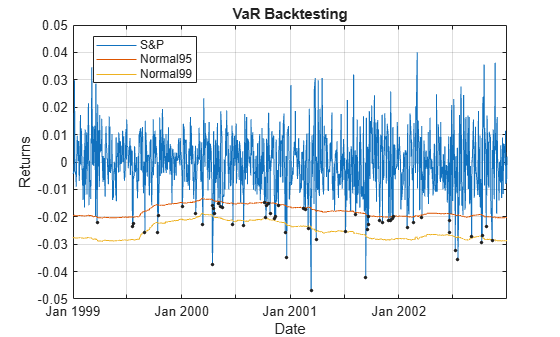# VaR Backtesting Workflow

This example shows a value-at-risk (VaR) backtesting workflow and the use of VaR backtesting tools. For a more comprehensive example of VaR backtesting, see Value-at-Risk Estimation and Backtesting.

### Step 1. Load the VaR backtesting data.

Use the `VaRBacktestData.mat` file to load the VaR data into the workspace. This example works with the `EquityIndex`, `Normal95`, and `Normal99` numeric arrays. These arrays are equity returns and the corresponding VaR data at 95% and 99% confidence levels is produced with a normal distribution (a variance-covariance approach). See Value-at-Risk Estimation and Backtesting for an example on how to generate this VaR data.

```load('VaRBacktestData') disp([EquityIndex(1:5) Normal95(1:5) Normal99(1:5)])```
``` -0.0043 0.0196 0.0277 -0.0036 0.0195 0.0276 -0.0000 0.0195 0.0275 0.0298 0.0194 0.0275 0.0023 0.0197 0.0278 ```

The first column shows three losses in the first three days, but none of these losses exceeds the corresponding VaR (columns 2 and 3). The VaR model fails whenever the loss (negative of returns) exceeds the VaR.

### Step 2. Create a `varbacktest` object.

Create a `varbacktest` object for the equity returns and the VaRs at 95% and 99% confidence levels.

```vbt = varbacktest(EquityIndex,[Normal95 Normal99],... 'PortfolioID','S&P', ... 'VaRID',{'Normal95' 'Normal99'}, ... 'VaRLevel',[0.95 0.99],'Time', Date); disp(vbt)```
``` varbacktest with properties: PortfolioData: [1043x1 double] VaRData: [1043x2 double] Time: [1043x1 datetime] PortfolioID: "S&P" VaRID: ["Normal95" "Normal99"] VaRLevel: [0.9500 0.9900] ```

### Step 3. Generate a VaR backtesting plot.

Use the `plot` function to visualize the VaR backtesting data. This type of visualization is a common first step when performing a VaR backtesting analysis.

```plot(vbt) ylabel("Returns") xlabel("Date")```### Step 4. Run a summary report.

Use the `summary` function to obtain a summary for the number of observations, the number of failures, and other simple metrics.

`summary(vbt)`
```ans=2×10 table PortfolioID VaRID VaRLevel ObservedLevel Observations Failures Expected Ratio FirstFailure Missing ___________ __________ ________ _____________ ____________ ________ ________ ______ ____________ _______ "S&P" "Normal95" 0.95 0.94535 1043 57 52.15 1.093 58 0 "S&P" "Normal99" 0.99 0.9837 1043 17 10.43 1.6299 173 0 ```

### Step 5. Run all tests.

Use the `runtests` function to display the final test results all at once.

`runtests(vbt)`
```ans=2×11 table PortfolioID VaRID VaRLevel TL Bin POF TUFF CC CCI TBF TBFI ___________ __________ ________ ______ ______ ______ ______ ______ ______ ______ ______ "S&P" "Normal95" 0.95 green accept accept accept accept accept reject reject "S&P" "Normal99" 0.99 yellow reject accept accept accept accept accept accept ```

### Step 6. Run individual tests.

After running all tests, you can investigate the details of particular tests. For example, use the `tl` function to run the traffic light test.

`tl(vbt)`
```ans=2×9 table PortfolioID VaRID VaRLevel TL Probability TypeI Increase Observations Failures ___________ __________ ________ ______ ___________ _______ ________ ____________ ________ "S&P" "Normal95" 0.95 green 0.77913 0.26396 0 1043 57 "S&P" "Normal99" 0.99 yellow 0.97991 0.03686 0.26582 1043 17 ```

### Step 7. Create VaR backtests for multiple portfolios.

You can create VaR backtests for different portfolios, or the same portfolio over different time windows using the `select` function. Run tests over two different subwindows of the original test window.

```Ind1 = year(Date)<=2000; Ind2 = year(Date)>2000; vbt1 = select(vbt, ... 'TimeRange', Ind1, ... 'NewPortfolioID', 'S&P, 1999-2000'); vbt2 = select(vbt, ... 'TimeRange', Ind2, ... 'NewPortfolioID', 'S&P, 2001-2002');```

### Step 8. Display a summary report for both portfolios.

Use the `summary` function to display a summary for both portfolios.

```Summary = [summary(vbt1); summary(vbt2)]; disp(Summary)```
``` PortfolioID VaRID VaRLevel ObservedLevel Observations Failures Expected Ratio FirstFailure Missing ________________ __________ ________ _____________ ____________ ________ ________ ______ ____________ _______ "S&P, 1999-2000" "Normal95" 0.95 0.94626 521 28 26.05 1.0749 58 0 "S&P, 1999-2000" "Normal99" 0.99 0.98464 521 8 5.21 1.5355 173 0 "S&P, 2001-2002" "Normal95" 0.95 0.94444 522 29 26.1 1.1111 35 0 "S&P, 2001-2002" "Normal99" 0.99 0.98276 522 9 5.22 1.7241 45 0 ```

### Step 9. Run all tests for both portfolios.

Use the `runtests` function to display the final test result for both portfolios.

```Results = [runtests(vbt1);runtests(vbt2)]; disp(Results)```
``` PortfolioID VaRID VaRLevel TL Bin POF TUFF CC CCI TBF TBFI ________________ __________ ________ ______ ______ ______ ______ ______ ______ ______ ______ "S&P, 1999-2000" "Normal95" 0.95 green accept accept accept accept accept reject reject "S&P, 1999-2000" "Normal99" 0.99 green accept accept accept accept accept accept accept "S&P, 2001-2002" "Normal95" 0.95 green accept accept accept accept accept accept accept "S&P, 2001-2002" "Normal99" 0.99 yellow accept accept accept accept accept accept accept ```

### Step 10. List exceptions.

Use the `exceptions` function to list all the exceptions in the full test window. An exception is a data point where `-portfolioValue` > `threshold`*`varValue`, a loss is defined as `-portfolioValue` for a given point in time, and the `SeverityRatio` is the ratio `Loss`/`VaR`.

```exceptionsTable = exceptions(vbt); head(exceptionsTable)```
``` Time Loss Normal95 SeverityRatio ___________ ________ ________ _____________ 23-Mar-1999 0.022232 0.020108 1.1057 20-Jul-1999 0.023592 0.01998 1.1808 22-Jul-1999 0.022426 0.019963 1.1234 31-Aug-1999 0.02561 0.017744 1.4433 13-Oct-1999 0.025715 0.015562 1.6525 15-Oct-1999 0.019577 0.015711 1.2461 04-Jan-2000 0.016179 0.014776 1.0949 18-Feb-2000 0.018806 0.014471 1.2996 ```